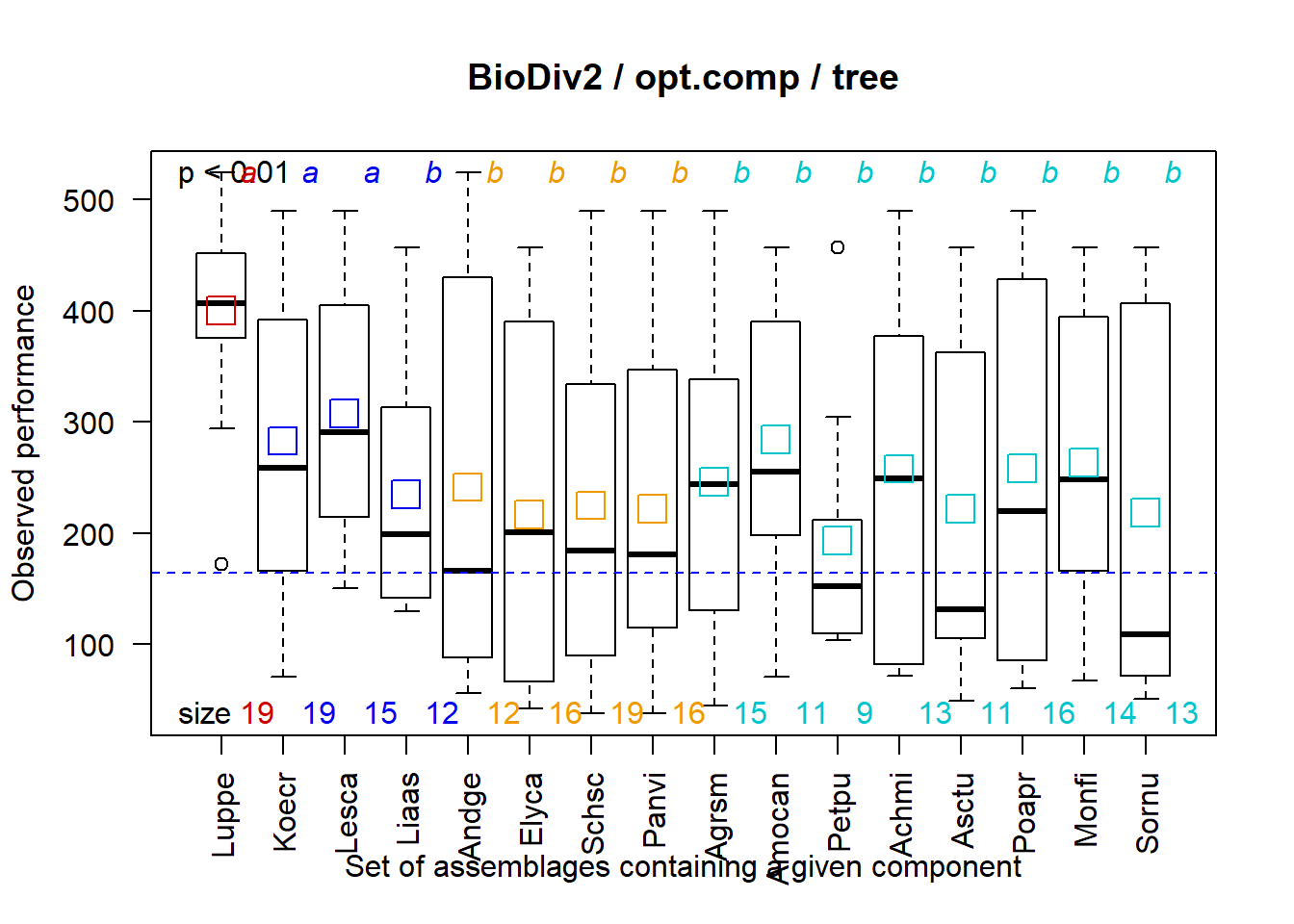# The options of fclust_plot

#### 2020-11-30

The function fclust_plot can conveniently plot the primary and secondary trees of component clustering, observed, modelled and predicted performances of assemblages, composition and mean performances of assembly motifs, mean performances of assemblages containing a given components, or performances of some given assemblages.

## The option opt.tree

opt.tree manages the plot of primary and secondary trees of component clustering. The option appears as a list of sub-options : opt.tree = list(“cal”, “prd”, “leg”, cols, “zoom”, window, “all”).

• “cal” plots the primary tree of component clustering. A blue dashed line indicates the optimum number of component clusters. A red dashed line indicates the value of tree efficiency.

• “prd” plots the validated, secondary tree of component clustering. A red dashed line indicates the value of tree efficiency.

• “leg” : if “cal” or “prd” are checked, the option leg plots the content in components of validated clusters of the secondary tree.

• cols gives a priori defined colours to each component. This option is useful to identify some given components, or components a priori clustered, into an a posteriori clustering.

• “zoom” : if “cal” or “prd” are checked, the option “zoom” allows to only plot the first, significant component clusters. window = n (n = number of components to plot) must then be informed.

• “all” is equivalent to opt.tree = list(“cal”, “prd”, “leg”).

``````apriori <- c("F","C3","L","C4","F","C3","C3","L","F","L","F","C4","L","C3","C4","C4")
res <- fclust(dat.2004, nbElt, opt.mod = "byelt", opt.mean = "gmean")

fclust_plot(res, main = "BioDiv2", opt.tree = list("prd"))
fclust_plot(res, main = "BioDiv2", opt.tree = list("prd", cols = apriori))``````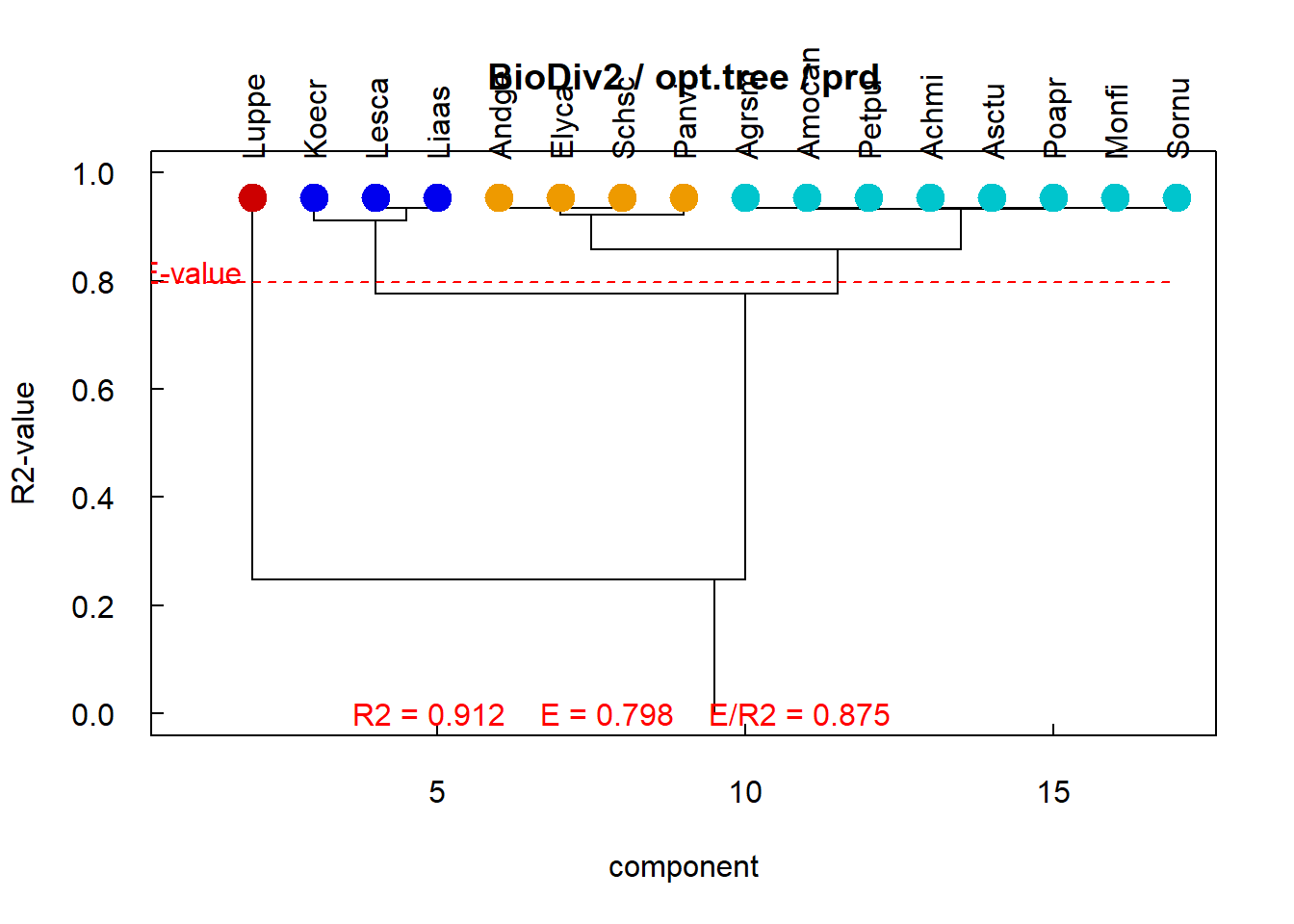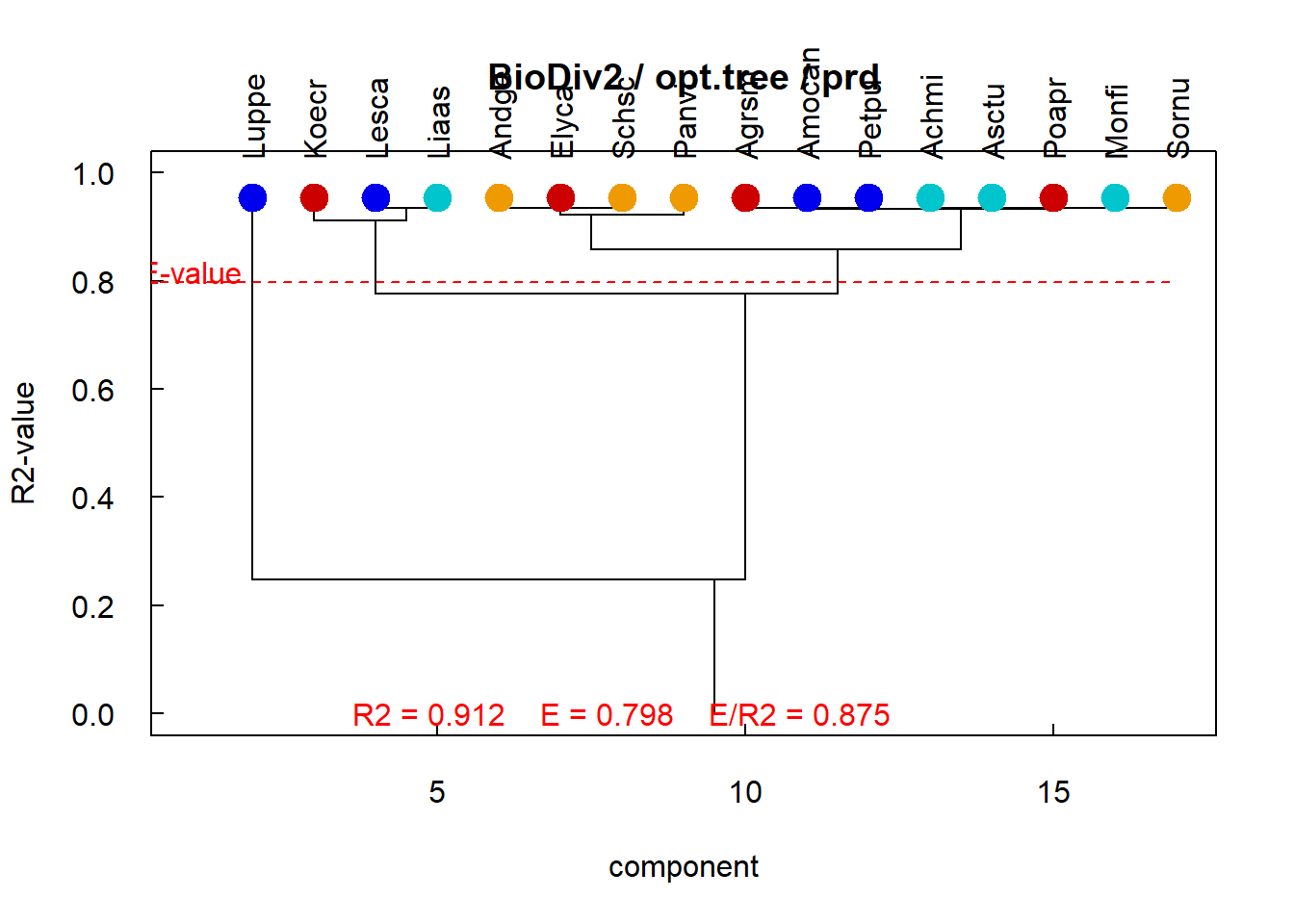Opt.tree manages the plot of primary and secondary trees

Both the graphs show the validated, secondary tree pruned above the optimum number of component clusters. However, on left graph components belonging to a cluster are of same colour, on right graph components belonging to clusters a priori defined by the user are of same colour. Here, the meadow species are a priori clustered in Legumes (in red), Forbs (in cyan), C3-grasses (in blue) and C4 grasses (in gold). The x-axis of tree is the system components. The y-axis of tree is the quality criterion of modelling, i.e. the coefficient of determination R2. Modelling efficiency (E-value) is indicated by a red dashed line. Values of R2, E and E/R2 are indicated.

## The option opt.perf

opt.perf manages the plot of observed, modelled and predicted performances of assemblages, and associated statistics. The option appears as a list of sub-options : opt.perf = list(“stats_I”, “stats_II”, “cal”, “prd”, “pub”, “missing”, “calprd”, “seq”, “ass”, “aov”, pvalue, “all”).

• “stats_I”, “stats_II” plot the statistics associated to fit of primary and secondary trees.
``fclust_plot(res, main = "BioDiv2", opt.perf = list("stats_II"))``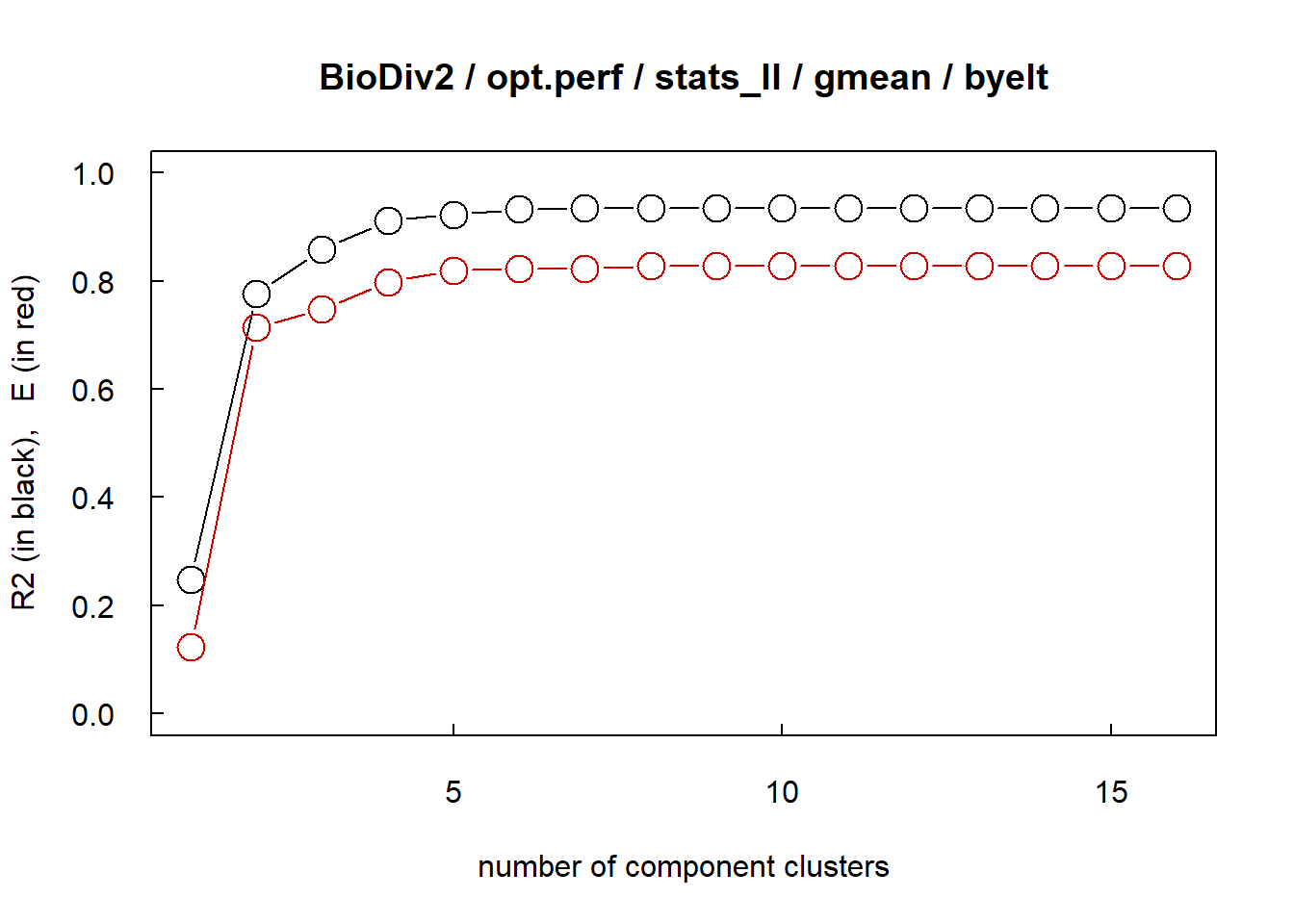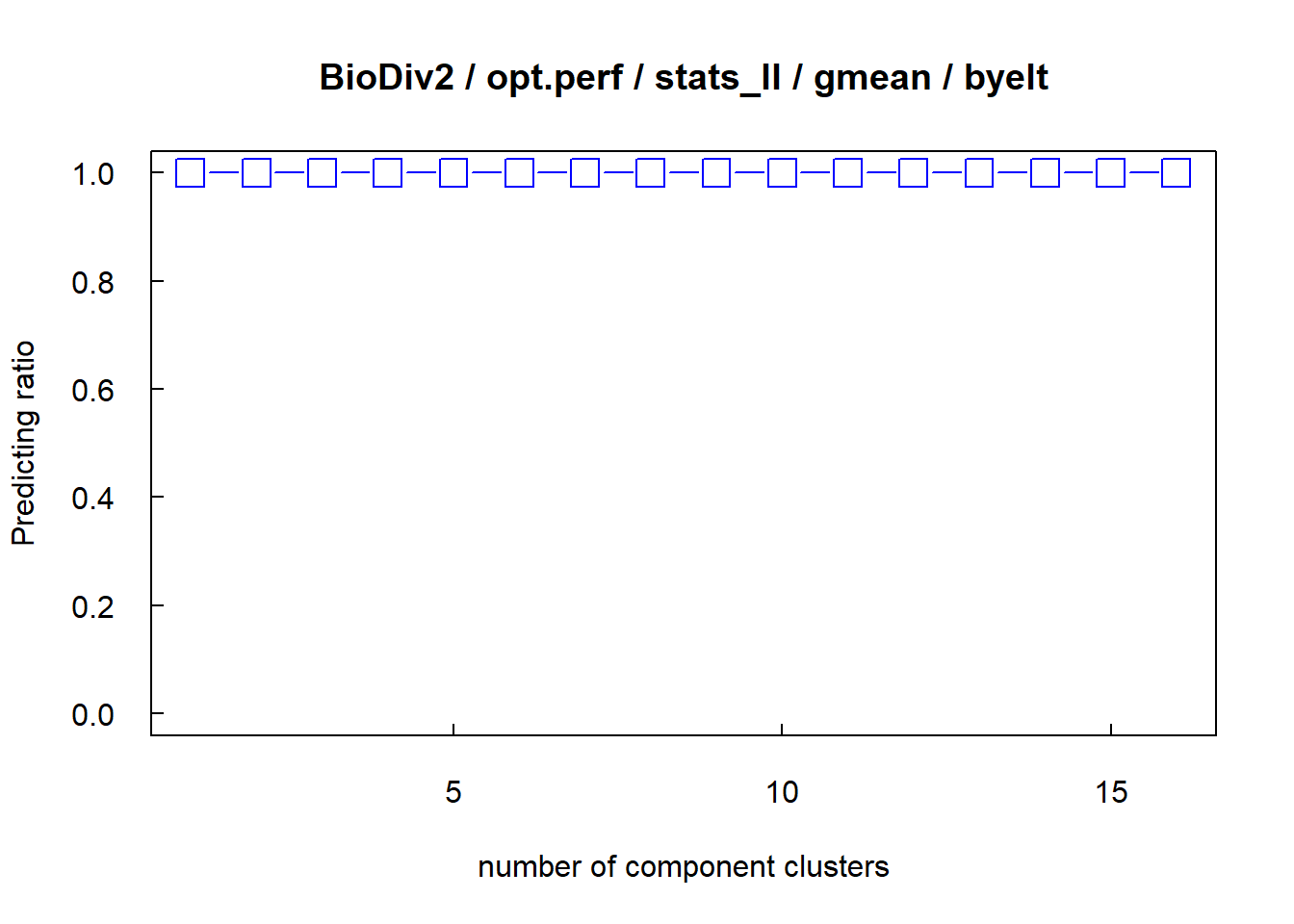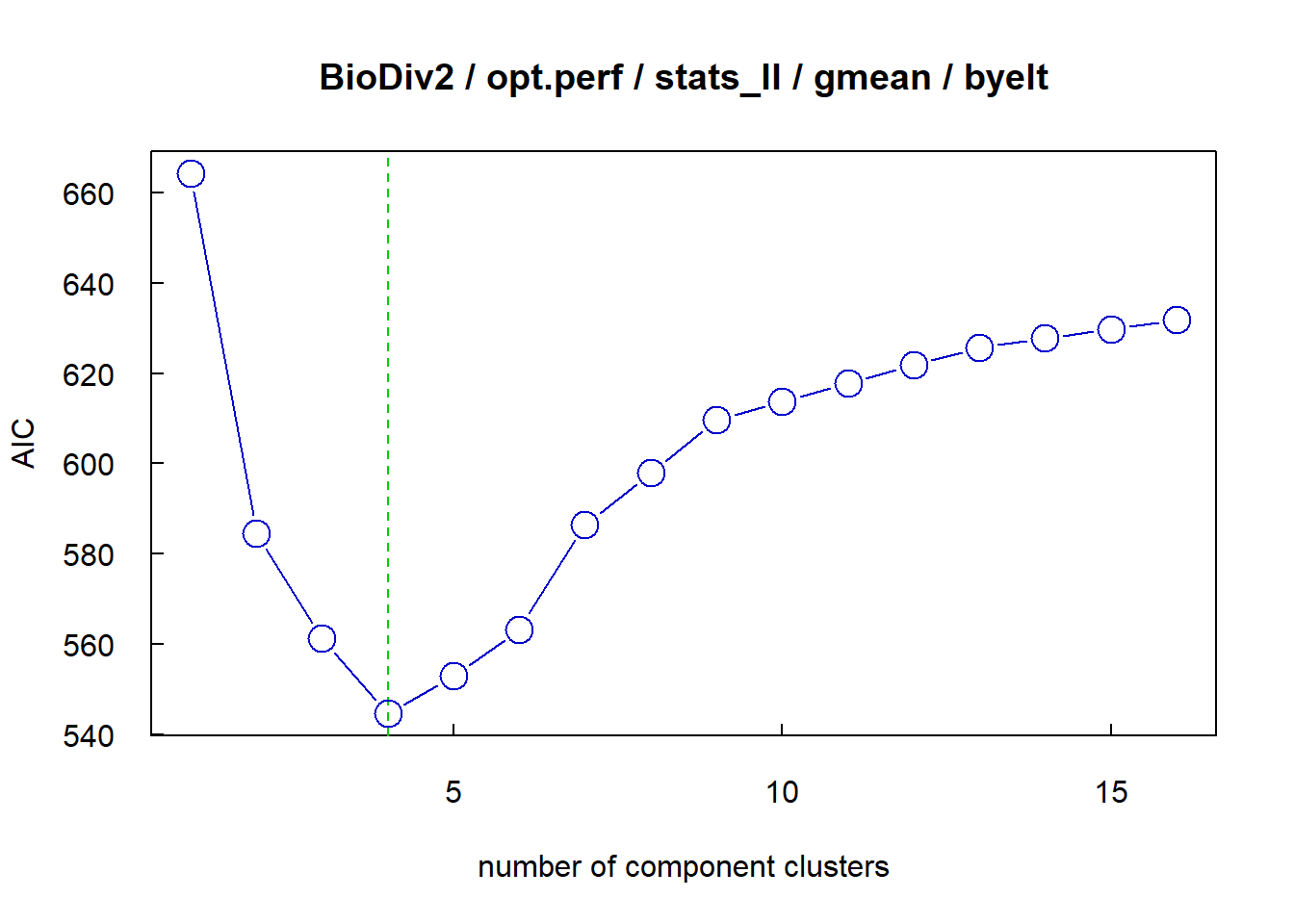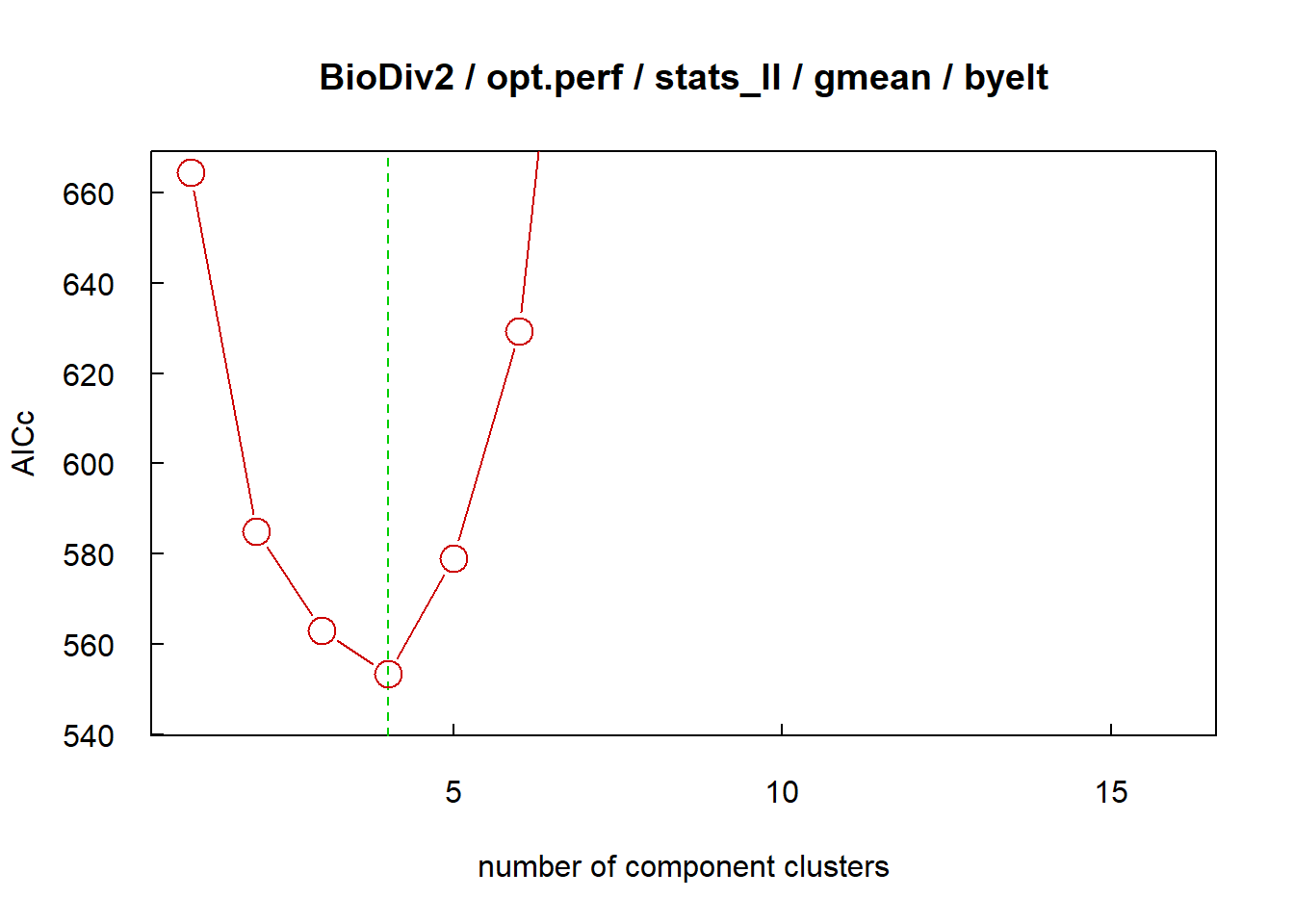Opt.perf=list(‘stats_xx’) manages the plot of statistics

The first left graph shows coefficient of determination R2 (black circles) and efficiency E (red circles) of validated secondary. The right graph shows the proportion of assemblages of which the performance cannot be predicted by cross-validation only using the clustering model (blue squares). Here all performances are predicted by using the whole secondary tree. Both the lowest graphs show AIC (blue circles) and AICc (red circles) of models. Green dashed line indicates the optimum number of component clusters.

• “cal”, “prd”, “pub” : “cal” plots modelled performance versus observed performance, “prd” plots performances modelled and predicted by cross-validation versus observed performance, “pub” plots performances modelled and predicted by cross-validation versus observed performance using only one colour and symbol, which is useful for publication.
``fclust_plot(res, main = "BioDiv2", nbcl = 3, opt.perf = list("prd", "aov", pvalue = 0.01, "pub"))``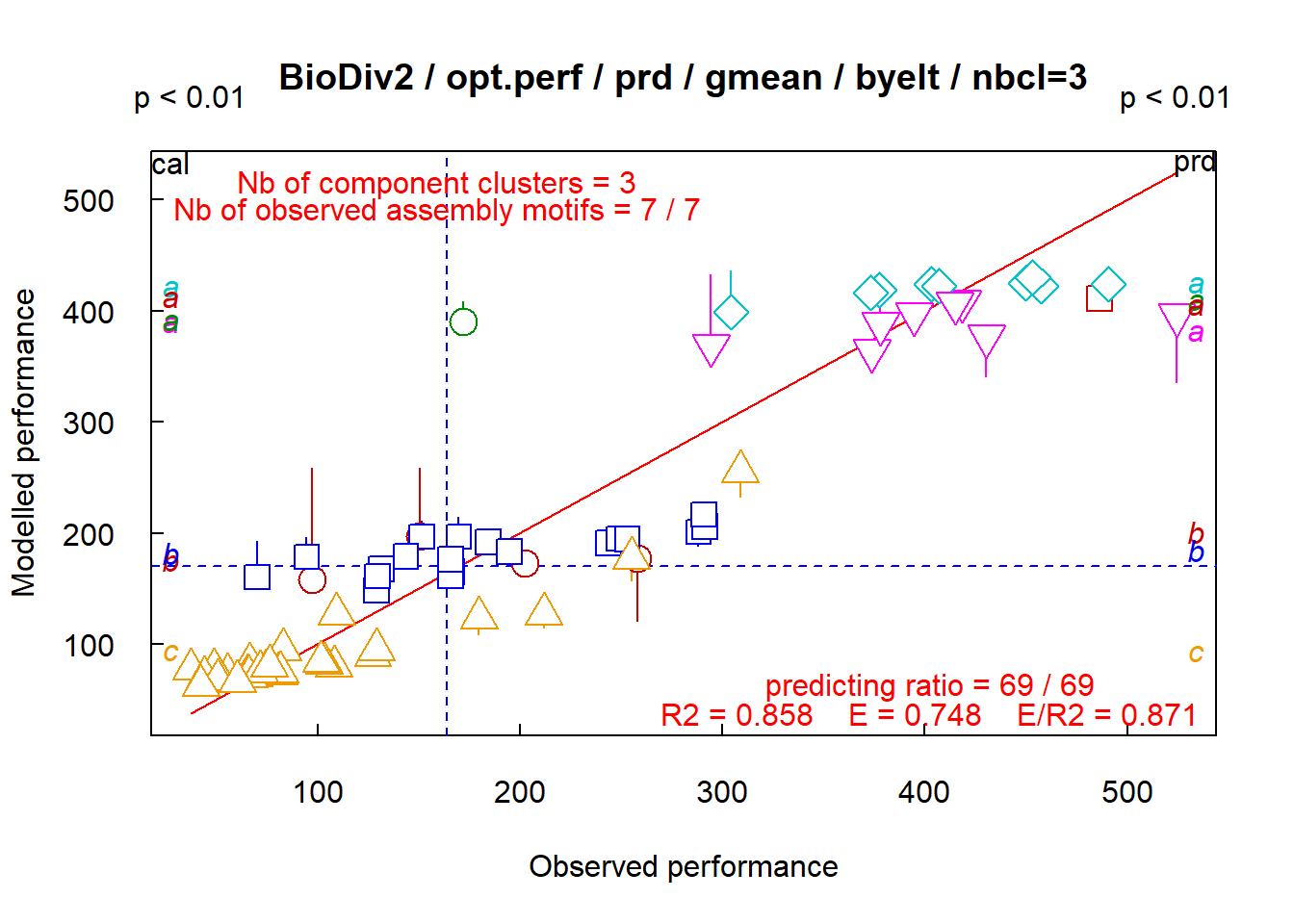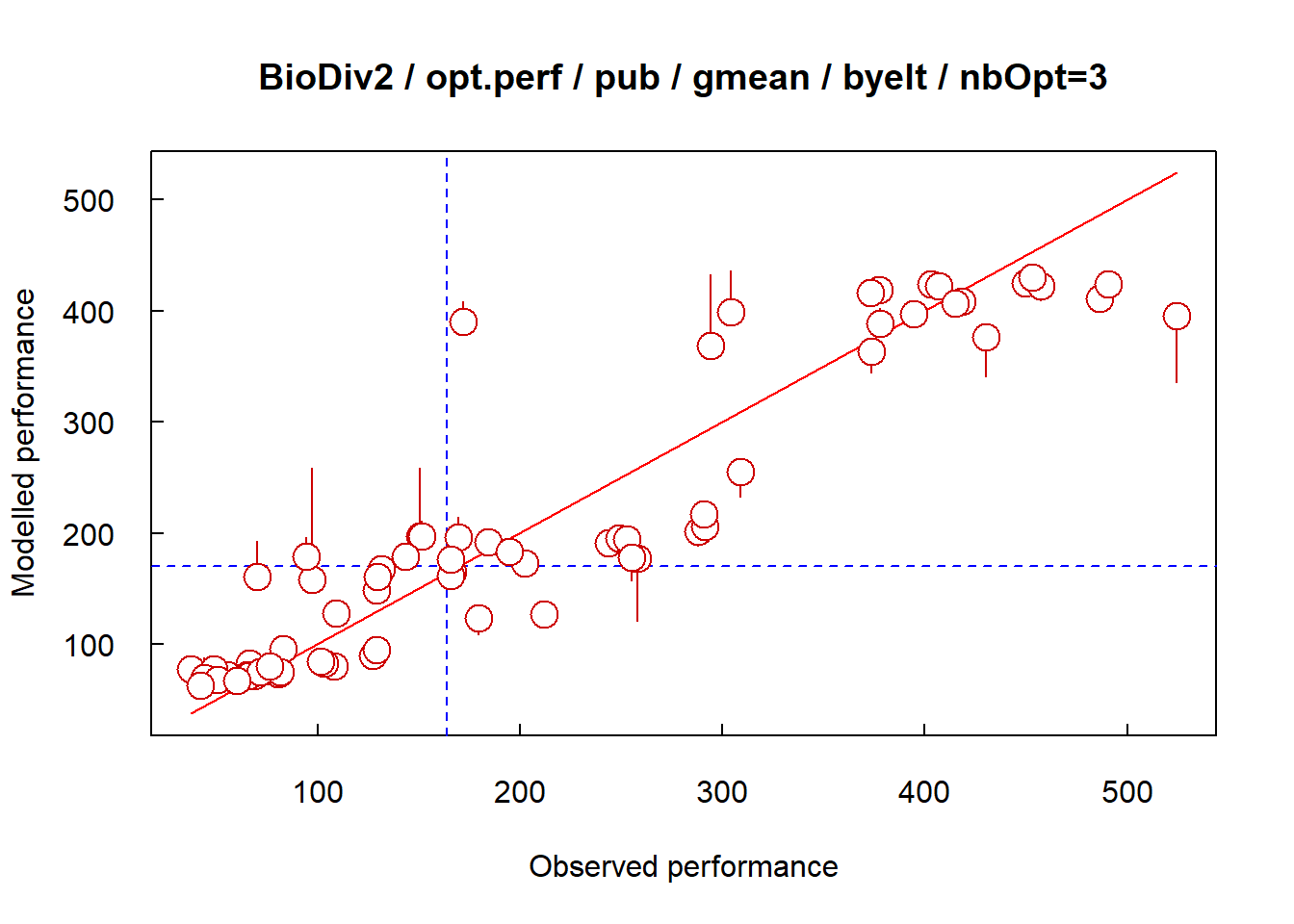Opt.perf=list(‘prd’) on left, =list(‘pub’) on right

Both graphs show the model goodness-of-fit, left and right graphs differing only by the used colours and symbols. On left graph, the performances of assemblages belonging to different assembly motifs are plotted with different colours and symbols. The symbols correspond to performances predicted by the model (modelled performances), of which the goodness-of-fit is evaluated by tree coefficient of determination R2. The vertical lines correspond to performances independently predicted by cross-validation (independently predicted performances), of which the quality is evaluated by tree efficiency E. Global statistic are indicated (number of component clusters, number of assembly motifs, R2, E and the ratio E/R2). A variance analysis is indicated on detailled graph, on the left side for modelled performances, on the right side for performances predicted by cross-validation, using a pvalue = 0.01. On both graphs, the solid red line is line 1:1, and the blue dashed lines are mean observed and modelled performances.

• “missing” identifies the assemblages of which the performance cannot be predicted using the clustering model of the current level.

• “calprd” plots performances predicted by cross-validation versus performances predicted by clustering model (“modelled performances”).

``````fclust_plot(res, main = "BioDiv2", nbcl = 4, opt.perf = list("missing"))
fclust_plot(res, main = "BioDiv2", nbcl = 4, opt.perf = list("calprd", "aov", pvalue = 0.01))``````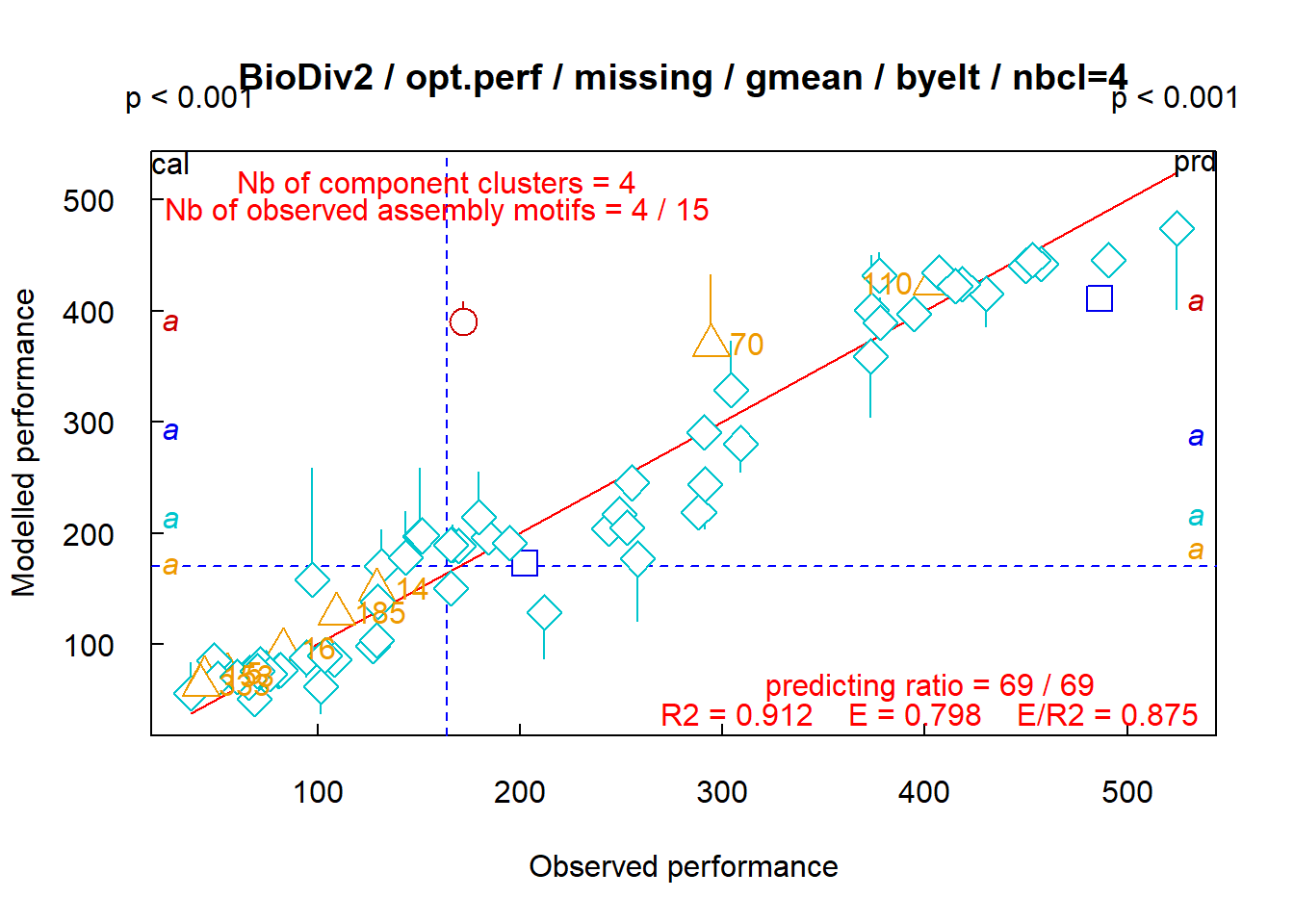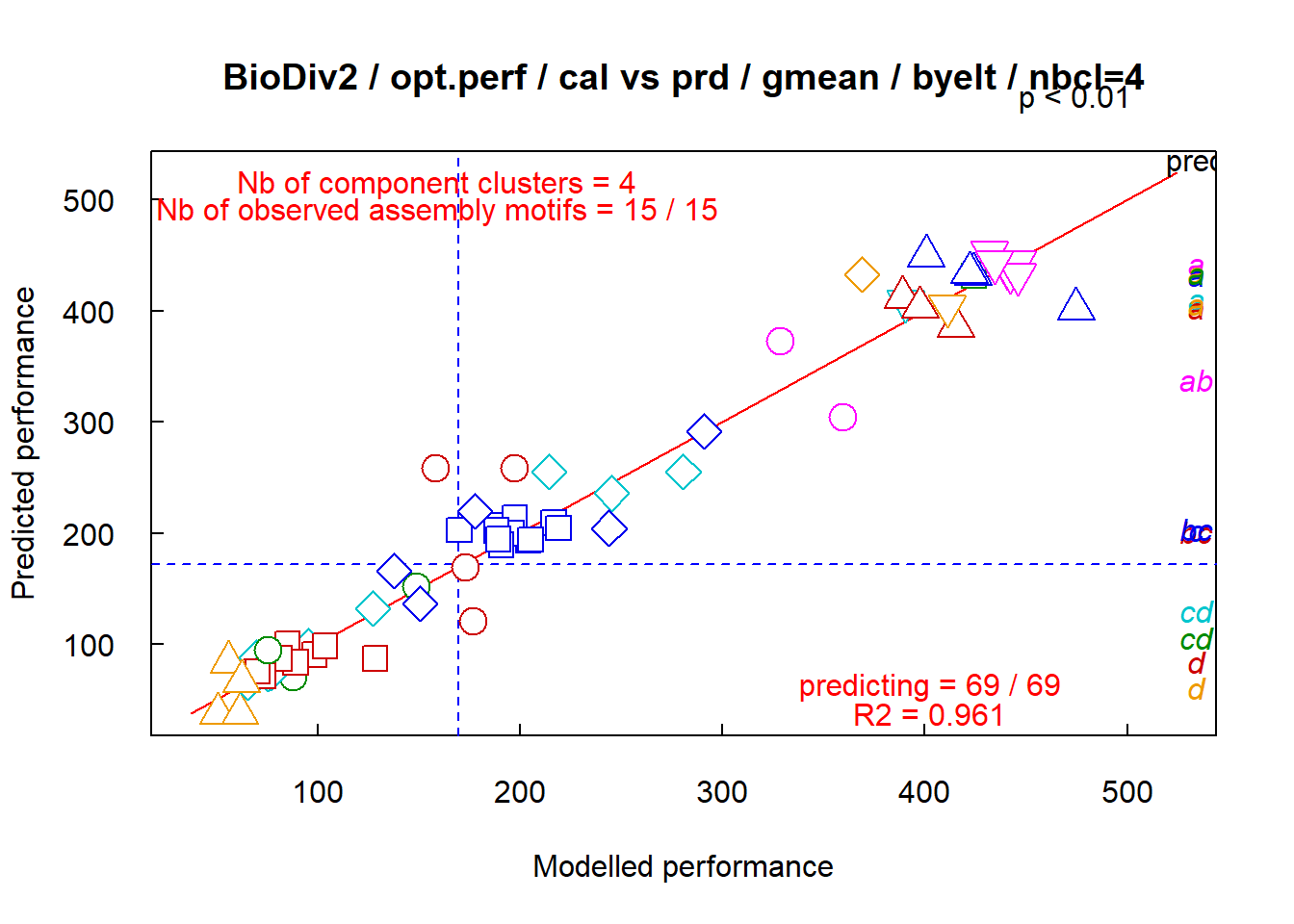Opt.perf=list(‘missing’) on left, =list(‘calprd’) on right

On the left graph, we observe that the performances of all assemblages can be predicted using nbcl = 4 clusters of components, except the assemblages “110”, “211” and “237”. On the right graph, we observe that the performances of all assemblages are as well predicted as modelled, except those of 3 assemblages (2 plotted as red circle, and one as gold square).

The right graph shows predicted performances versus modelled performances.

• “seq” plots performances of assembly motifs, from 1 to the optimum number of component clusters.
``fclust_plot(res, main = "BioDiv2", opt.perf = list("prd", "seq", "aov", pvalue = 0.01))``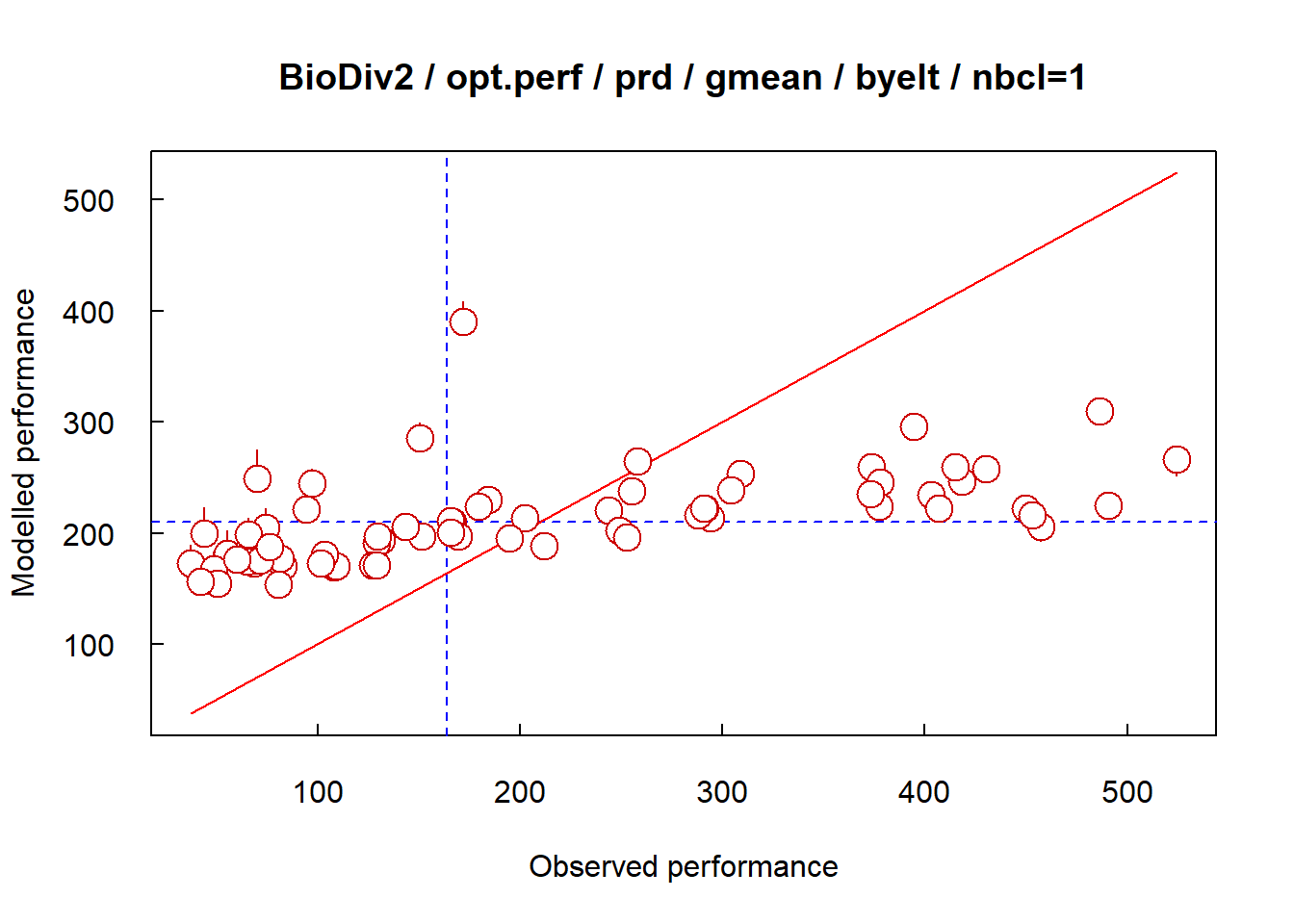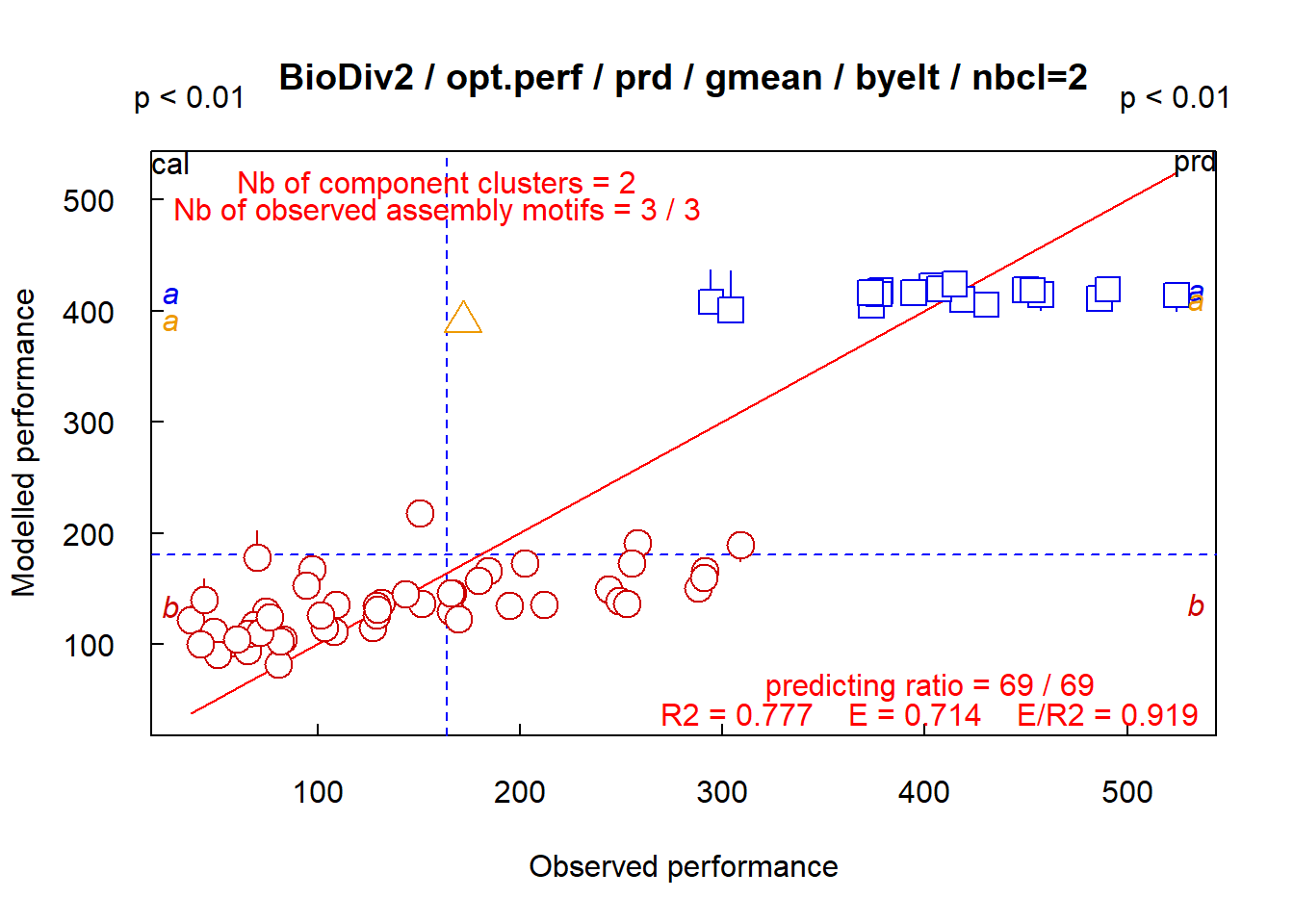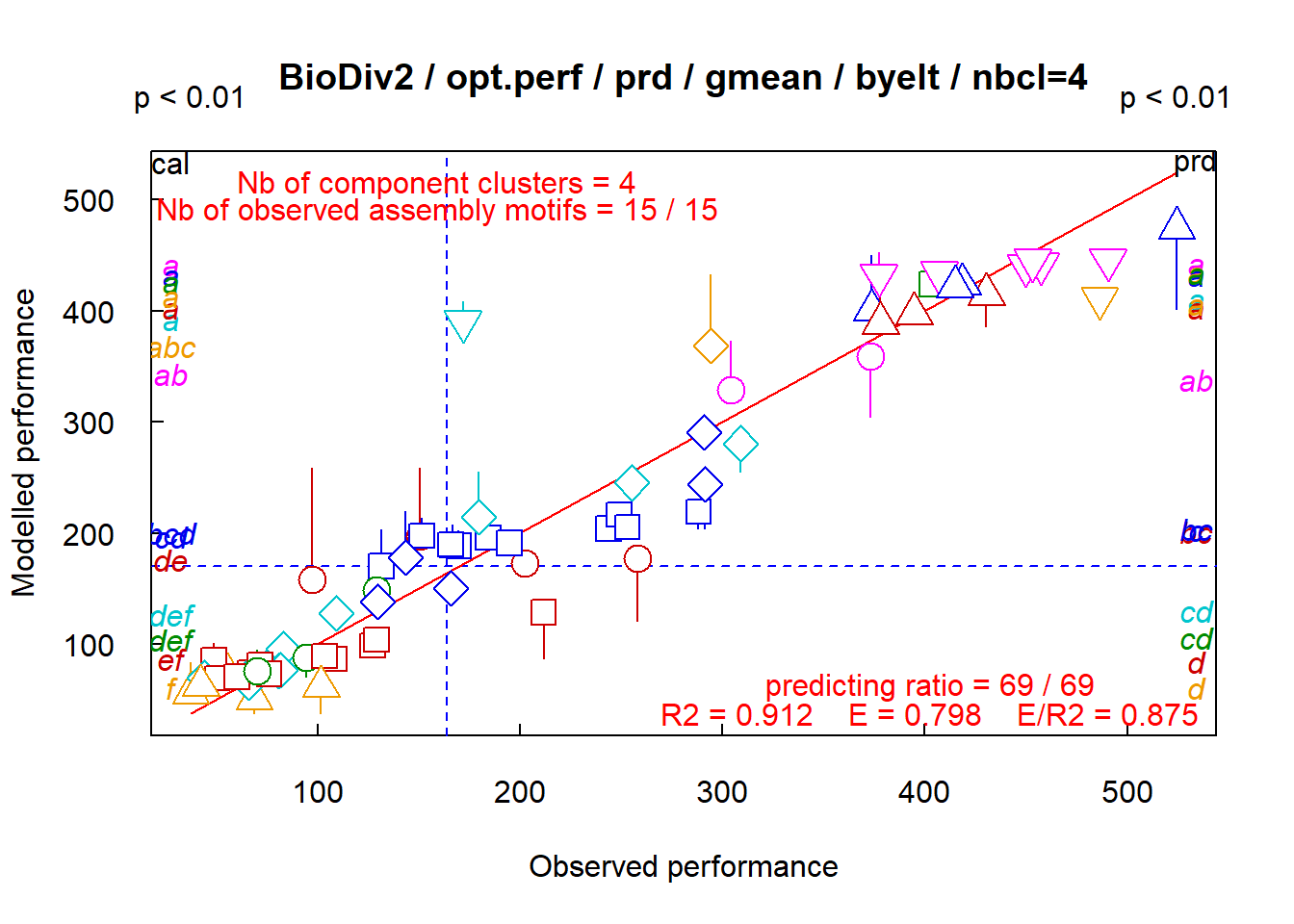Opt.perf=list(‘prd’, ‘seq’) plots performances predicted from1 to nbOpt component clusters

The number m of assembly motifs generates by the combination of nbcl component clusters is m = 2^nbcl - 1. It is 1, 3, 7, 15 and 31 for 1, 2, 3, 4 and 5 clusters. Here the optimum number of clusters is 4, and 13 among 15 assembly motifs are observed in the experiment.

• “ass” plots the name of each assemblage close to its performance.
``````fclust_plot(res, main = "BioDiv2", opt.perf = list("prd", "aov", pvalue = 0.01))
fclust_plot(res, main = "BioDiv2", opt.perf = list("prd", "ass"))``````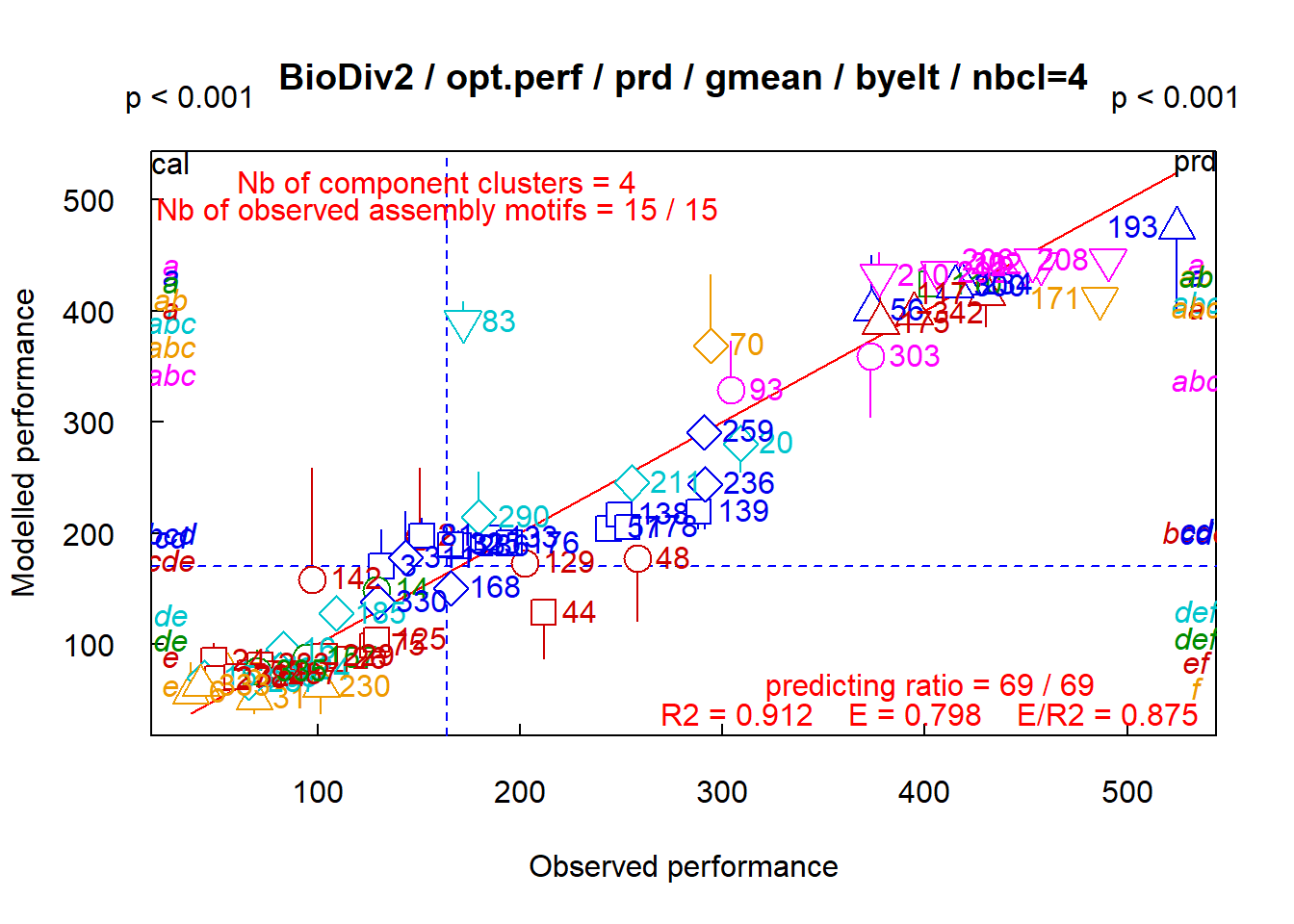Opt.perf=list(‘prd’, ‘ass’) adds the names of assemblages on plot

• “aov” does a variance analysis of assemblage performances by assembly motifs, and plot the results. pvalue = val (val = threshold of probability) must then be informed. If not, pvalue = 0.05 by default.

• “all” is equivalent to opt.perf = list(“cal”, “prd”, “pub”, “calprd”, “aov”, pvalue = 0.05).

## The option opt.ass

opt.ass plot different performances of some given, identified assemblages. The option is activated only when an assemblage performance is observed over several experiments (see vignette The option xpr : multi-functionality in functClust ).

## The option opt.motif

opt.motif manages the plot of mean performances of assembly motifs as boxplots. The option is a list, that can include opt.motif = list(“obs”, “cal”, “prd”, cols, “hor”, “seq”, pvalue, “all”):

• “obs”, “cal”, “prd” plot the observed, modelled or predicted by cross-validation mean performances of assembly motifs as boxplots. Assembly motifs are named as the combinations of component clusters.

• “hor” plot boxplots as horizontal boxes, that is x-axis corresponds to assemblage performances, and y-axis corresponds to assembly motifs.

• “seq” plots mean performances of assembly motifs, from 2 to the optimum number of component clusters.

• “aov” does a variance analysis of assemblage performances by assembly motifs, and plot the results. pvalue = val (val = threshold of probability) must then be informed. If not, pvalue = 0.05 by default.

• “all” is equivalent to opt.motif = list(“obs”, “cal”, “prd”, “seq”, “aov”, pvalue = 0.05).

``fclust_plot(res, main = "BioDiv2", opt.motif = list("prd", "hor", "seq", "aov", pvalue = 0.01))``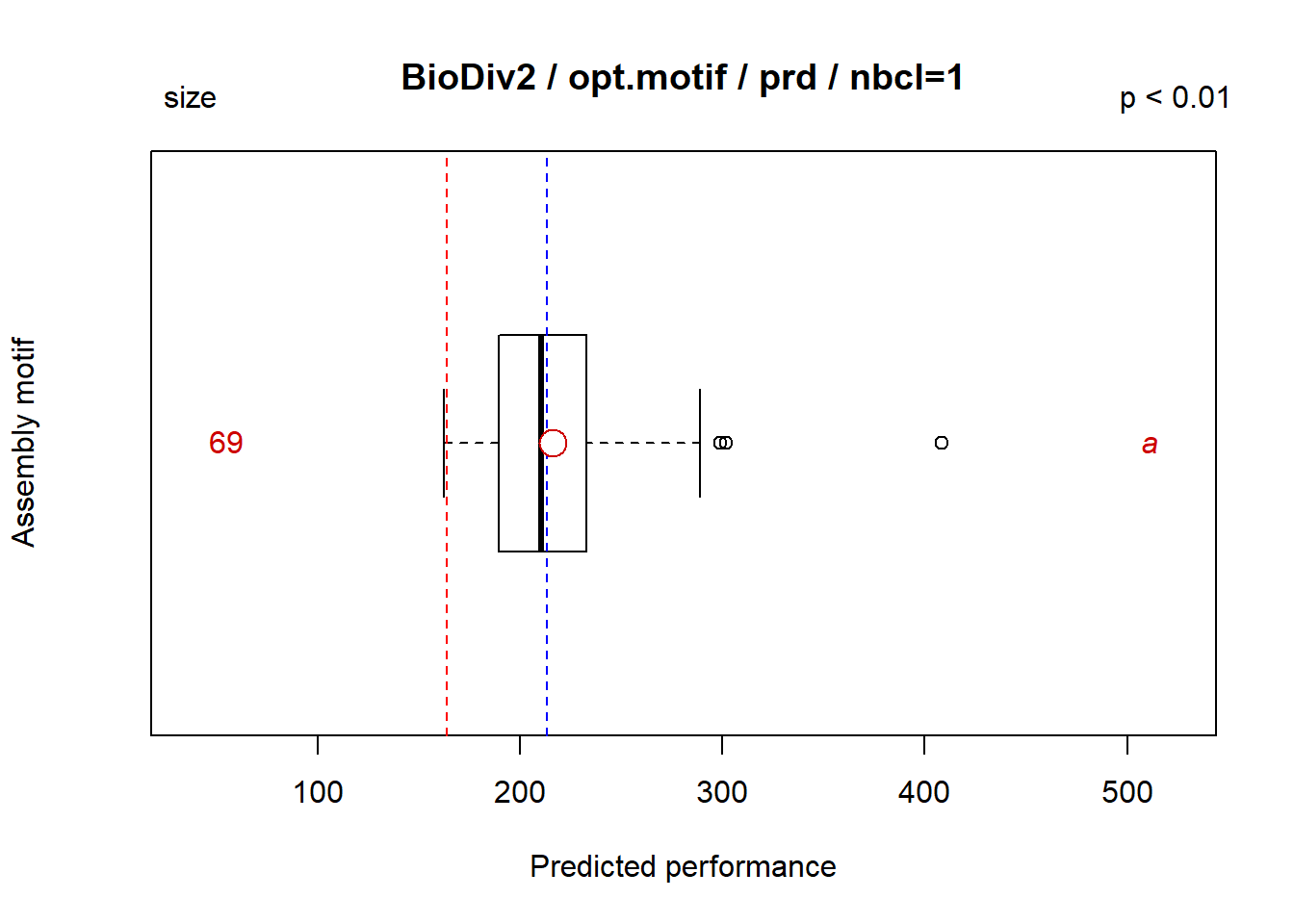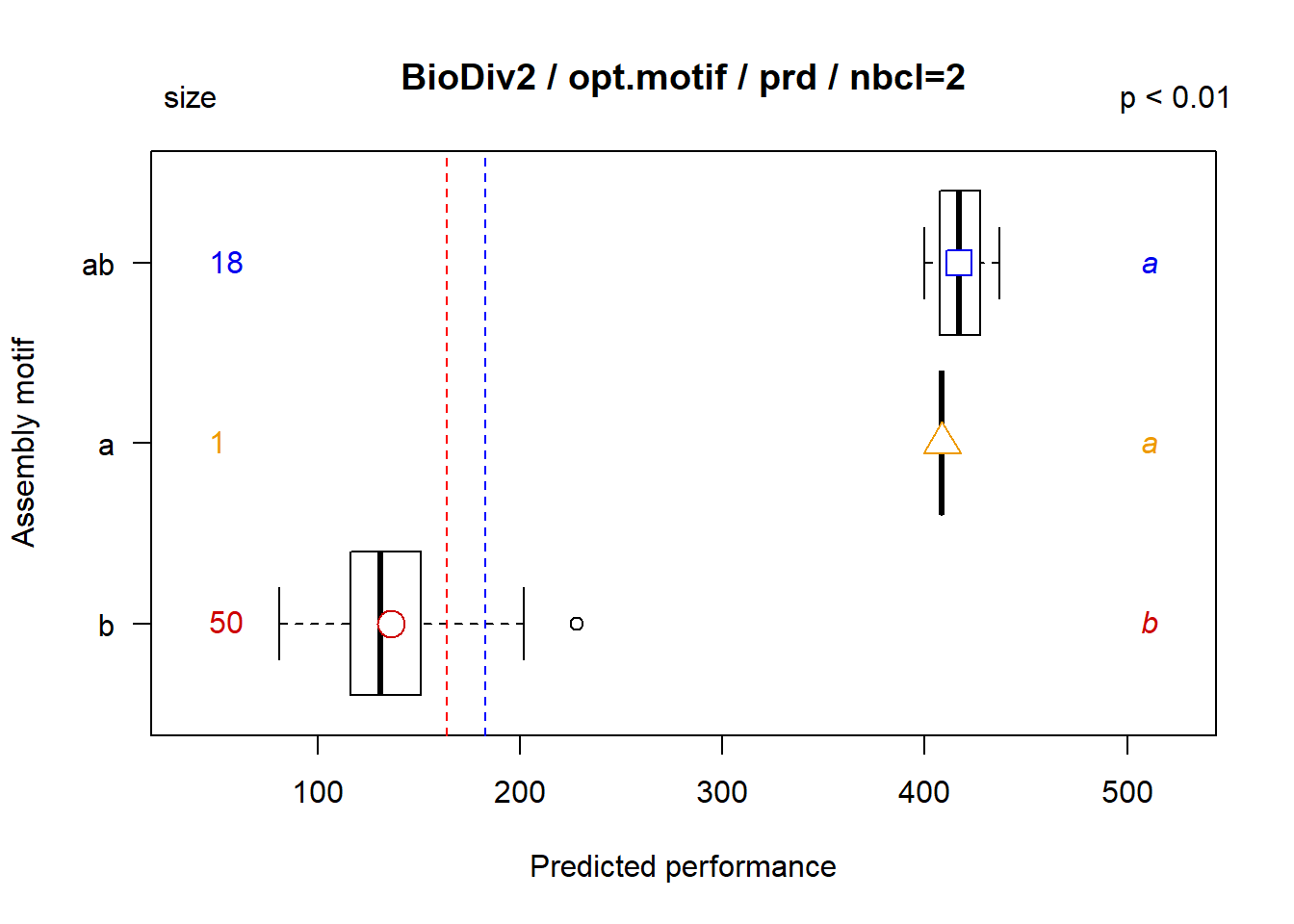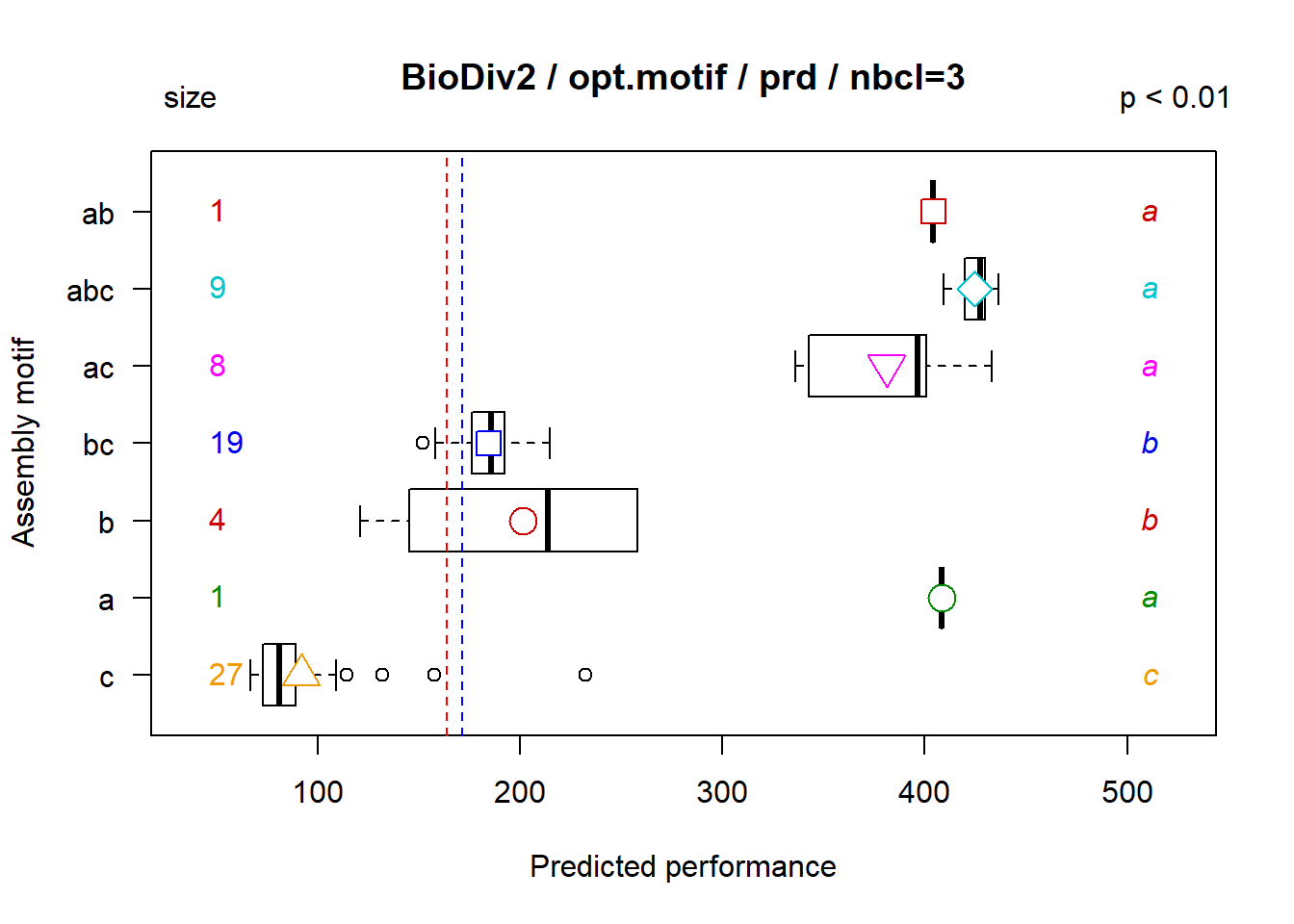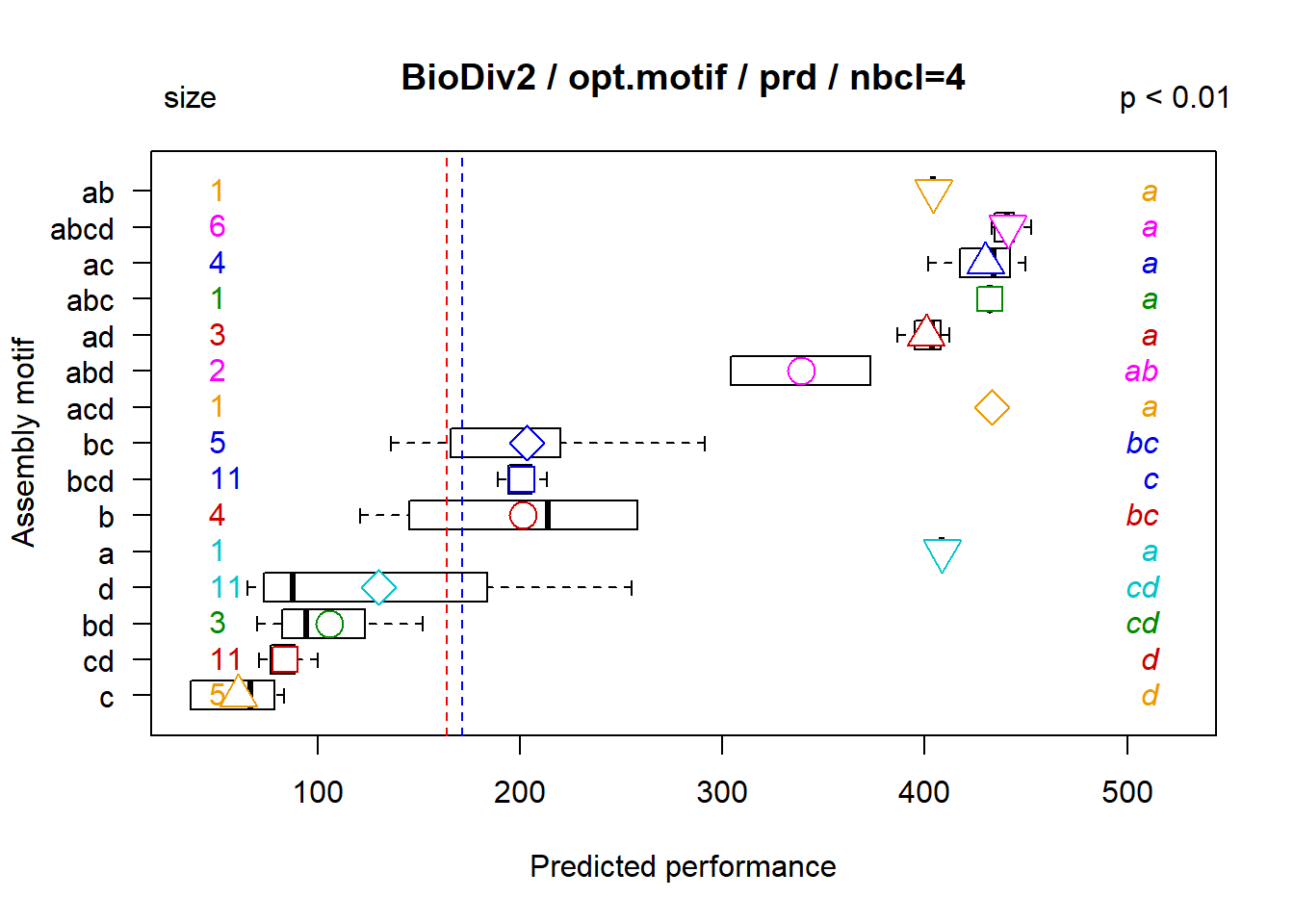Opt.motif=list(‘obs’, ‘seq’) plots performances sorted by assembly motif

The boxplots show observed performances of assemblages belonging to different assembly motifs. Means are indicated with different symbols, of same shape and colour as those used in opt.perf. The size (in number of assemblages) of each assembly motifs is indicated on the left side of the graph, and the results of a variance analysis of observed performances by assembly motif is indicated on the right side of the graph. The blue dashed lines are mean modelled performances (opt.motif = “prd”) and red dashed lines are mean observed performances.

## The option opt.comp

opt.comp manages the plot as boxplot of observed mean performances of assemblages that contain a given component. The option can include opt.comp = list(“tree”, “perf”, “hor”, cols, pvalue, “zoom”, window, “all”):

• “tree”, “perf” plot the observed mean performances of assemblages that contain a given component as boxplots, sorted like the clustering tree or sorted by increasing mean performance.

• “hor” plot boxplots as horizontal boxes, that is x-axis corresponds to assemblage performances, and y-axis corresponds to assembly motifs.

• cols allows to identify specified components, for instance a priori clustering into an a posteriori clustering.

• “aov” does a comparison od means between performance of set of assemblages that contain a given component against performance of set of other assemblages that do not contain the given component in consideration. Each compoent is analised separately. The result is “a” if performance of assemblage set is significantly higher, “b” if performance of assemblage set is not different, and “c” if performance of assemblage set is significantly lower, than performance of set of other assemblages that do not contain the given component. pvalue = val(val = threshold of probability) must then be informed. If not, pvalue = 0.05 by default.

• “all” is equivalent to opt.comp = list(“tree”, “aov”, pvalue = 0.05, “zoom”, window = 20).

``fclust_plot(res, main = "BioDiv2", opt.comp = list("tree", "leg", "aov", pvalue = 0.01))``